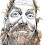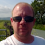## Sunday, 29 May 2016

### Divide and Conquer Line Algorithm for the ZX Spectrum

While attempting to write a game in 256 bytes I needed a routine to draw lines, but Bresenham's line algorithm weighs in at approx ~120 bytes. The only suitable alternative I'm aware of is recursive divide and conquer: divide a line into two smaller lines and call the draw routine with each in turn:

``````/* Draw a line from (ax,ay) to (bx,by) */

int draw ( ax, ay, bx, by )
{
int midx, midy;
midx = ( ax+bx ) / 2;
midy = ( ay+by ) / 2;
if ( midx != ax && midy != ay )
{
draw( midx, midy, ax, ay );
draw( bx, by, midx, midy );
plot( midx, midy );
}
}
``````

This is significantly smaller thank Bresenham's, 32 byte of Z80. However, there are a couple of compromises: it's slower and the lines aren't perfect because the rounding errors accumulate.

``````; draw lines using recursive divide and conquer
; from de = end1 (d = x-axis, e = y-axis)
; to   hl = end2 (h = x-axis, l = y-axis)

DRAW:
call PLOT

push hl

; calculate hl = centre pixel

ld a,l
rra
ld l,a
ld a,h
rra
ld h,a

; if de (end1) = hl (centre) then we're done

or a
sbc hl,de
jr z,EXIT

ex de,hl
call DRAW    ; de = centre, hl = end1
ex (sp),hl
ex de,hl
call DRAW    ; de = end2, hl = centre

ex de,hl
pop de
ret

EXIT:
pop hl
ret

; ---------------------------

; plot d = x-axis, e = y-axis

PLOT:
push hl
ld a,d
and 7
ld b,a
inc b
ld a,e
rra
scf
rra
or a
rra
ld l,a
xor e
and 248
xor e
ld h,a
ld a,l
xor d
and 7
xor d
rrca
rrca
rrca
ld l,a
ld a,1
PLOTBIT:
rrca
djnz PLOTBIT
or (hl)
ld (hl),a
pop hl
ret
``````

Alternatively the `de(end1) = hl(centre)` test can be replaced with a recursion depth count to create an even slower 28 byte routine:

``````; draw lines using recursive divide and conquer
; from de = end1 (d = x-axis, e = y-axis)
; to   hl = end2 (h = x-axis, l = y-axis)

DRAW:
ld c,8

DRAW2:
dec c
jr z,EXIT

push de

; calculate de = centre pixel

ld a,l
rra
ld e,a
ld a,h
rra
ld d,a

call DRAW2   ; de = centre, hl = end1
ex (sp),hl
call DRAW2   ; de = centre, hl = end2

call PLOT
ex de,hl
pop hl
EXIT:
inc c
ret
``````1.Fantastic work! Thanks for sharing, if I had a ZX Spectrum, I'd definitely try this out. :)

2.Hi John,

Do you know where I can find an implementation of the Bresenham line drawing in Z80? I'm not too bothered about the size of the code, I'm just trying to understand how to do it correctly, :)

3.That PLOT: routine pretty much sums up why I never managed to learn machine code. It's easy to learn what all the instructions do on their own, but when they get put together, they just become a jumble.

You set the last three bits of the X co-ordinate and add one to it, for some reason. And then it gets really weird when you move on to the Y coordinate. I think "rra, scf, rra, or a, rra" might mean something like "divide by 8 then add 64" but when you Xor it with the number you first thought of, then And it with something else, and Xor it with the number you first thought of once again, my brain just turns to mush.

How does anyone follow all that, let alone understand it enough to write it?

1.Ah, I've just worked out that setting the last 3 bits and adding one is probably like rounding the X co-ordinate up to the next multiple of 8. Maybe that's something to do with the X axis having 8 pixels to each screen byte? Am I getting warmer?

Though if you're trying to use as few bytes as possible (to pack as much game as possible into a 16K Spectrum?) a simple CALL to ROM would only be 3 bytes. That's a lot less than all this "Round up and divide by the number you first thought of" stuff.